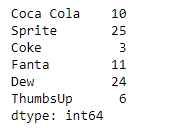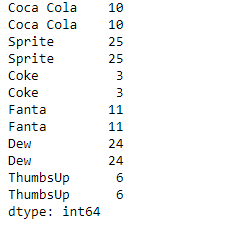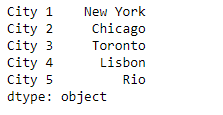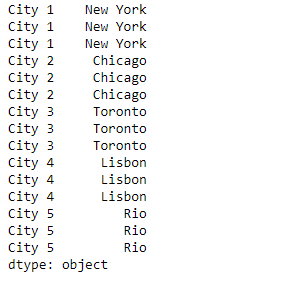Python | Pandas Series.repeat()

• Last Updated : 10 Feb, 2019

Pandas series is a One-dimensional ndarray with axis labels. The labels need not be unique but must be a hashable type. The object supports both integer- and label-based indexing and provides a host of methods for performing operations involving the index.

Pandas Series.repeat() function repeat elements of a Series. It returns a new Series where each element of the current Series is repeated consecutively a given number of times.

Syntax: Series.repeat(repeats, axis=None)

Parameter :
repeats : The number of repetitions for each element.
axis : None

Returns : repeated_series

Example #1: Use Series.repeat() function to repeat each value in the given Series object 2 times.

 # importing pandas as pdimport pandas as pd  # Creating the Seriessr = pd.Series([10, 25, 3, 11, 24, 6])  # Create the Indexindex_ = ['Coca Cola', 'Sprite', 'Coke', 'Fanta', 'Dew', 'ThumbsUp']  # set the indexsr.index = index_  # Print the seriesprint(sr)

Output :Now we will use Series.repeat() function to repeat each value of the given series object 2 times.

 # repeat twiceresult = sr.repeat(repeats = 2)  # Print the resultprint(result)

Output :As we can see in the output, the Series.repeat() function has returned a new series object where each values are repeated the specified number of times.

Example #2 : Use Series.repeat() function to repeat each value in the given Series object 3 times.

 # importing pandas as pdimport pandas as pd  # Creating the Seriessr = pd.Series(['New York', 'Chicago', 'Toronto', 'Lisbon', 'Rio'])  # Create the Indexindex_ = ['City 1', 'City 2', 'City 3', 'City 4', 'City 5']   # set the indexsr.index = index_  # Print the seriesprint(sr)

Output :Now we will use Series.repeat() function to repeat each value of the given series object 3 times.

 # repeat twiceresult = sr.repeat(repeats = 3)  # Print the resultprint(result)

Output :As we can see in the output, the Series.repeat() function has returned a new series object where each values are repeated the specified number of times.

My Personal Notes arrow_drop_up